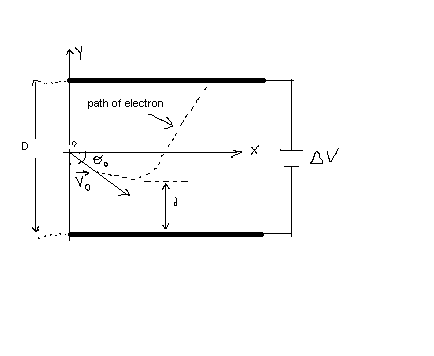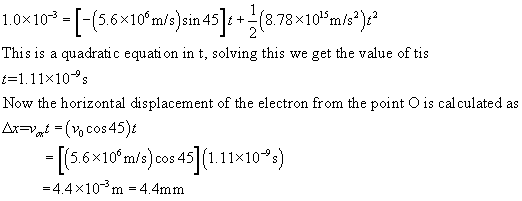Question# An electron is fired at a speed of v_0 = 5.6\times10^6 m/s and at an angle of \theta_0=–45^\circ between two parallel conductingplates that are D=2.0

Other
ANSWEREDAn electron is fired at a speed of $$\displaystyle{v}_{{0}}={5.6}\times{10}^{{6}}$$ m/s and at an angle of $$\displaystyle\theta_{{0}}=–{45}^{\circ}$$ between two parallel conductingplates that are D=2.0 mm apart, as in Figure. Ifthe potential difference between the plates is $$\displaystyle\triangle{V}={100}\ {V}$$, determine (a) how close d the electron will get to the bottom plate and (b) where the electron will strike the top plate.2021-05-14

Initial speed of the electron is $$\displaystyle{v}_{{0}}={5.6}\times{10}^{{6}}$$ m/s and $$\displaystyle\theta_{{0}}=-{45}^{\circ}$$
The distance betweeen two parallel conducting plates is $$\displaystyle{D}={2.0}\ {m}{m}={2.0}\cdot{10}^{{-{3}}}\ {m}$$
The potential difference between the plates is $$\displaystyle\triangle{V}={100}\ {V}$$
Now the direction of the electric field is along the Y-direction.
Now the electric field strength can be calculated by using the formula
$$\displaystyle{E}_{{y}}={\frac{{\triangle{V}}}{{{D}}}}={\frac{{{100}}}{{{2}\times{10}^{{-{3}}}}}}={5000}\ \frac{{N}}{{m}}$$
The acceleration of the electron is calculated as
$$\displaystyle{a}_{{y}}={\frac{{{F}_{{y}}}}{{{m}_{{e}}}}}={\frac{{{E}_{{y}}{q}}}{{{m}_{{e}}}}}$$
$$\displaystyle={\frac{{{\left({5000}\right)}{\left({1.6}\times{10}^{{-{19}}}\right)}}}{{{9.1}\times{10}^{{-{31}}}}}}={8.78}\times{10}^{{{15}}}\ \frac{{m}}{{s}^{{2}}}$$
(a) The initial velocity v0 can beresolved into two components.
Horizontal component is $$\displaystyle{v}_{{0}}{\cos{\theta}}$$
Vertical component is $$\displaystyle{v}_{{0}}{\sin{\theta}}$$
At the bottom plate the final velocity of the electron is zero.
That means $$\displaystyle{v}_{{y}}={0}$$
Now the vertical displacement of the electron is calculated byusing the formula
$$\displaystyle{{v}_{{y}}^{{2}}}={{v}_{{{0}{y}}}^{{2}}}+{2}{a}_{{y}}{\left(\triangle{y}\right)}$$
$$\displaystyle\triangle{y}={\frac{{-{{v}_{{{0}{y}}}^{{2}}}}}{{{2}{a}_{{y}}}}}$$
$$\displaystyle={\frac{{-{\left({v}_{{0}}{\sin{\theta}}\right)}^{{2}}}}{{{2}{a}_{{y}}}}}$$
$$\displaystyle={\frac{{-{\left[{\left(-{5.6}\times{10}^{{6}}\ \frac{{m}}{{s}}\right)}{\sin{{45}}}\right]}^{{2}}}}{{{2}{\left({8.78}\times{10}^{{{15}}}\right)}}}}$$
$$\displaystyle=-{0.89}$$ mm
Now the minimum distance above the bottom plate is then
$$\displaystyle{d}={\frac{{{D}}}{{{2}}}}+\triangle{y}={\frac{{{2.0}\times{10}^{{-{3}}}}}{{{2}}}}+{\left(-{0.89}{m}{m}\right)}={0.11}{m}{m}$$
b) The time for electron to travel from the point O to the upperplate is ccalculated as $$\displaystyle\triangle{y}={v}_{{{0}{y}}}{t}+{\frac{{{1}}}{{{2}}}}{a}_{{y}}{t}^{{2}}$$